# Number Grid: Adding and Subtracting Single Digit Numbers

Number Grid: Adding and Subtracting Single Digit Numbers• Here is a 100 number grid arranged in rows of 10.
• To add one to a number, we move one place to the right.
• For example, 15 + 1 = 16.
• To subtract one from a number we move one place to the left.
• For example 85 – 1 = 84.
We move right when adding and we move left when subtracting.

#### Printable Number Grid 1-100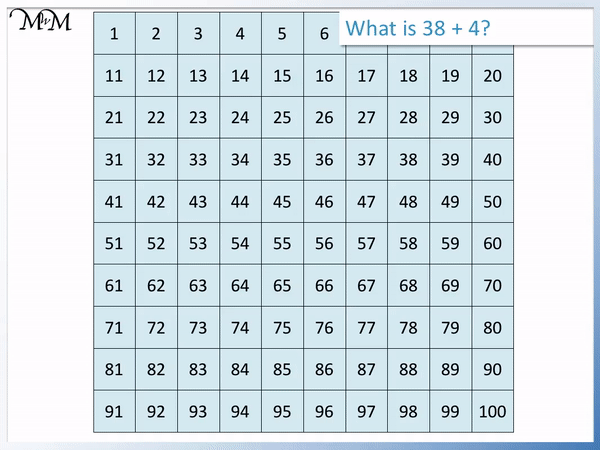• Every time we add 1, we move one place to the right on our number grid.
• When we reach the end of a , to add one more, we start at the beginning of the next row.
• 38 + 4 means that we find 38 and move to the right 4 places.
• We move one place to the right to get to 39.
• We move a second place to the right to get to 40.
• We are now at the end of the row so to add one a third time we start at the beginning of the next row at 41.
• We add one a fourth time to get to 42.
• We have added 4 in total by moving 4 places.
• 38 + 4 = 42.Supporting Lessons# How do we Add or Subtract Single-Digit Numbers using a 100 Number Grid?

When we add 1 to a number using the number grid, we move one place to the rightand when we subtract 1 from a number, we move one place to the left.

In this lesson, we will look at adding and subtracting multiples of 1 using the number grid.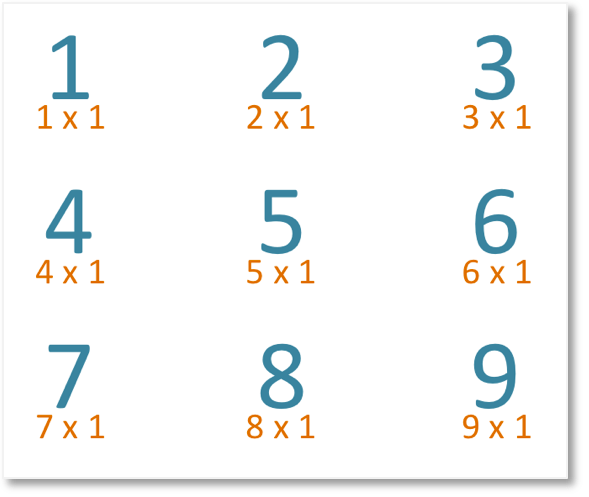Above are the first nine multiples of 1.

We can see that:

1 is 1 lot of 1

2 is 2lots of 1

3 is >3 lots of 1

4 is 4 lots of 1

5 is 5 lots of 1

6 is 6 lots of 1

7 is 7 lots of 1

8 is 8 lots of 1

9 is 9lots of 1

This tells us how many places we move left or right on the number grid.

For example, if we are adding 3, we move 3 places to the right on the number grid, or if we are subtracting 5, we move 5 places to the left on the number grid.

Here is an example of addition using the number grid: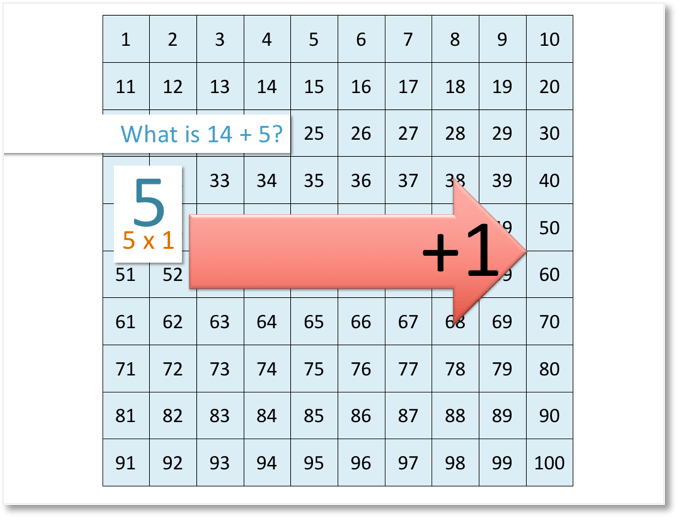We are asked: what is 14 + 5?

When we add 1, we move 1 place to the right on the number grid.

5 is 5 lots of 1, so we move 5 places to the right.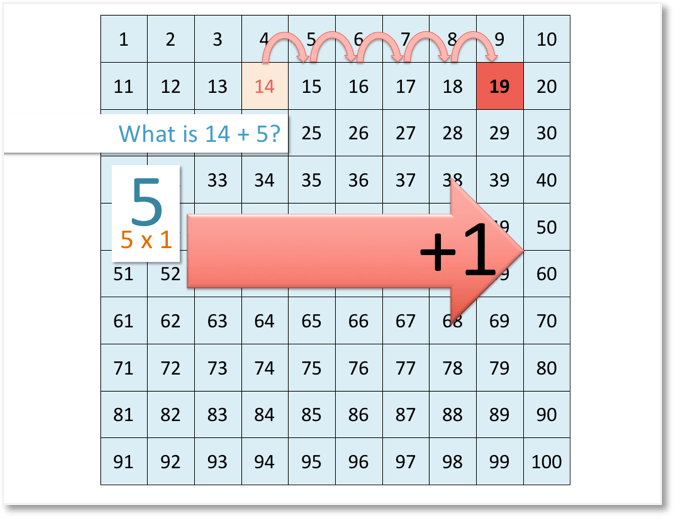We start at 14 and we move 5 places to the right on the number grid.

We stop at 19.

Therefore,

14 + 5 = 19.

Here is another example of addition using the number grid: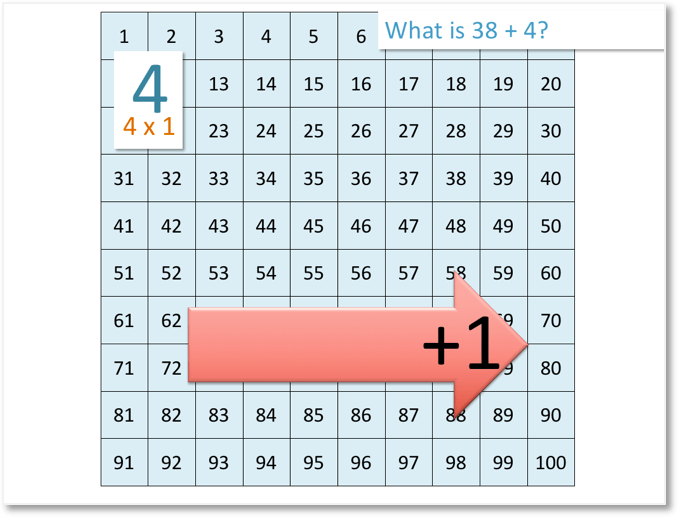We are asked: what is 38 + 4?

When we add 1, we move 1 place to the right on the number grid.

4 is 4 lots of 1, so we move 4 places to the right.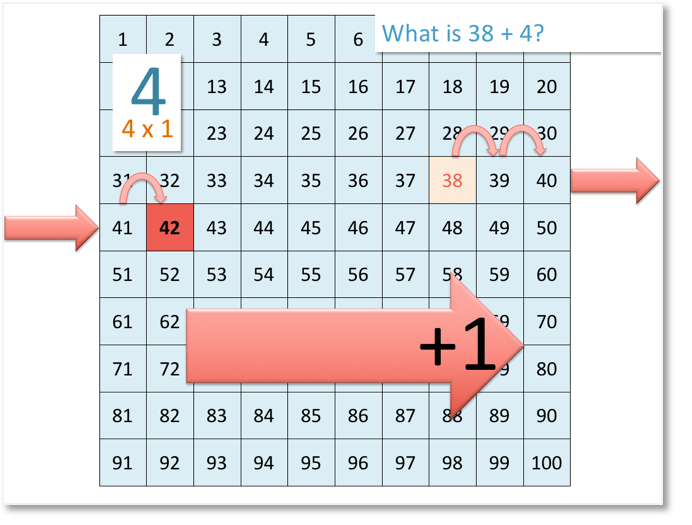We start at 38 and want to move 4 places to the right.

After moving 2 places, we land on 40, which is at the end of the row.

So, we can’t move any further to the right.

Our next step is to move to the beginning of the next row.

We have now moved 3 places. We now move 1 more place to the right.

In total, we have moved 4 places.

Therefore,

38 + 4 = 42.

Here is an example of subtraction using the number grid: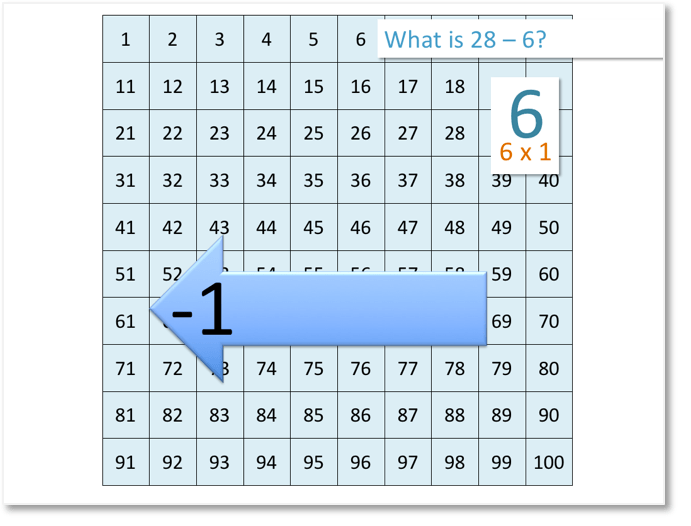We are asked: what is 28 – 6?

When we subtract 1, we move 1 place to the left on the number grid.

6 is 6 lots of 1, so we move 6 places to the left.We start at 28 and we move 6 places to the left on the number grid.

We stop at 22.

Therefore,

28 – 6 = 22.

Here is another example of subtraction using the number grid:We are asked: what is 91 – 8?

When we subtract 1, we move 1 place to the left on the number grid.

8 is 8 lots of 1, so we move 8 places to the left.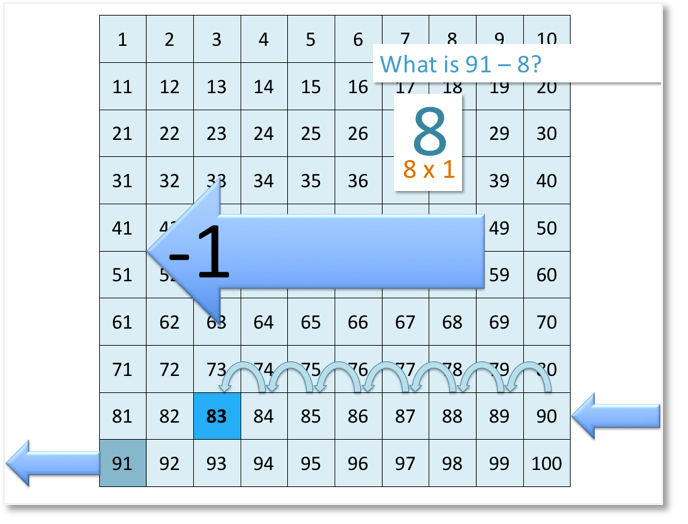We start at 91 and want to move 8 places to the left.

We are starting at the beginning of the row, so we can’t move any further to the left.

Therefore, our first step is to move to the end of the previous row.

We have moved 1 place, so we now need to move 7 more places to the left.

We stop at 83.

Therefore,

91 – 8 = 83.Now try our lesson Number Grid: Adding and Subtracting Ten, where we learn how to add and subtract ten using a 100 number grid.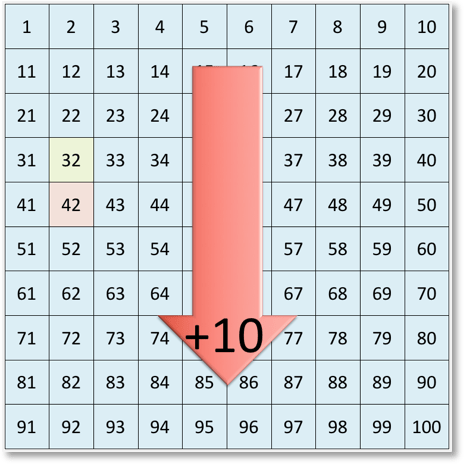error: Content is protected !!